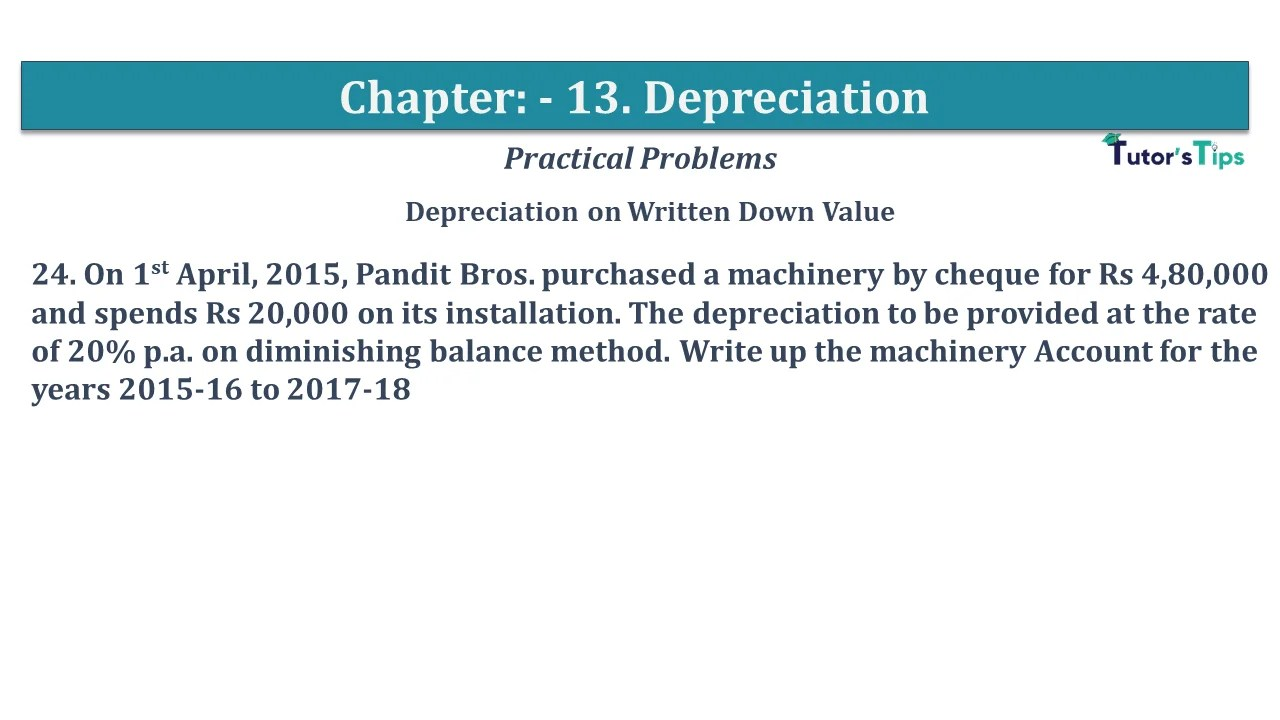# Question No 24 Chapter No 13 – USHA Publication 11 ClassQuestion No 24 Chapter No 13

Depreciation on Written Down Value

24. On 1st April, 2015, Pandit Bros. purchased a machinery by cheque for Rs 4,80,000 and spends Rs 20,000 on its installation. The depreciation to be provided at the rate of 20% p.a. on diminishing balance method. Write up the machinery Account for the years 2015-16 to 2017-18

The solution of Question No 24 Chapter No 13:-

 Dr. Machine A/c Cr. Date Particulars J.F. Amount Date Particulars J.F. Amount 01/04/15 To Cash A/c 5,00,000 31/03/16 By Deprecation A/c*1 1,00,000 31/03/16 By Balance C/d 4,00,000 5,00,000 5,00,000 01/04/16 To Balance b/d 4,00,000 31/03/17 By Depreciation A/c*2 80,000 31/03/17 By Balance C/d 3,20,000 4,00,000 4,00,000 01/04/17 To Balance b/d 3,20,000 31/03/18 By Deprecation A/c*3 64,000 31/03/19 8By Balance C/d 2,56,000 3,20,000 3,20,000

Note: The amount of depreciation have to charged for the year 2015-16 for the half the year to find the answer which given the in the book.

Working note:-

*1:- Calculation of the amount of Depreciation on furniture for the year 2015-16
Machinery purchased on 1st Apr 2015
Depreciation = Value of Asset X Rate of Depreciation X Period
Value of Asset = 5,00,000
Rate of Depreciation = 20%
Period = from 01/04/2015 to 31/03/2016 i.e. 12 months
(from the date of purchase/Beginning balance to the end of the financial year)
= 5,00,000 X 20/100 X 12/ 12
Depreciation = 1,00,000
Total Depreciation for the year = 1,00,000

*2:– Calculation of the amount of Depreciation on furniture for year 2016-17
Machinery purchased on 1st Apr 2015
Depreciation = Value of Asset X Rate of Depreciation X Period
Value of Asset = 4,00,000
Rate of Depreciation = 20%
Period = from 01/10/2016 to 31/03/2017 i.e. 12 months
(from the date of purchase/Beginning balance to end of the financial year)
= 4,00,000 X 20/100 X 12/ 12
Depreciation = 80,000
Total Depreciation for the year = 80,000

*3:- Calculation of the amount of Depreciation on furniture for the year 2018-19
Machinery purchased on 1st Apr 2015
Depreciation = Value of Asset X Rate of Depreciation X Period
Value of Asset = 3,20,000
Rate of Depreciation = 20%
Period = from 01/04/2017 to 31/03/2018 i.e. 12 months
(from the date of purchase/Beginning balance to the end of the financial year)
= 3,20,000 X 20/100 X 12/ 12
Depreciation = 64,000
Total Depreciation for the year = 64,000

Depreciation | Meaning | Methods | Examples

Comment if you have any question.

Also, Check out the solved question of all Chapters: –

Chapter No. 1 – Introduction

Chapter No. 2 – Theory Base of Accounting

Chapter No. 3 – Vouchers and transactions

Chapter No. 4 – Journal

Chapter No. 5 – Ledger

Chapter No. 6 – Cash Book

Chapter No. 7 – Other Subsidiary Books

Chapter No. 8 – Journal Proper

Chapter No. 9 – Trial Balance

Chapter No. 10 – Bank Reconciliation Statement

Chapter No. 11 – Depreciation

Chapter No. 12 – Provisions and Reserves

Chapter No. 13 – Bills of Exchange

Chapter No. 14 – Rectification of Errors

Chapter No. 15 – Financial Statements – (Without Adjustments)

Chapter No. 16 – Financial Statements – (With Adjustments)